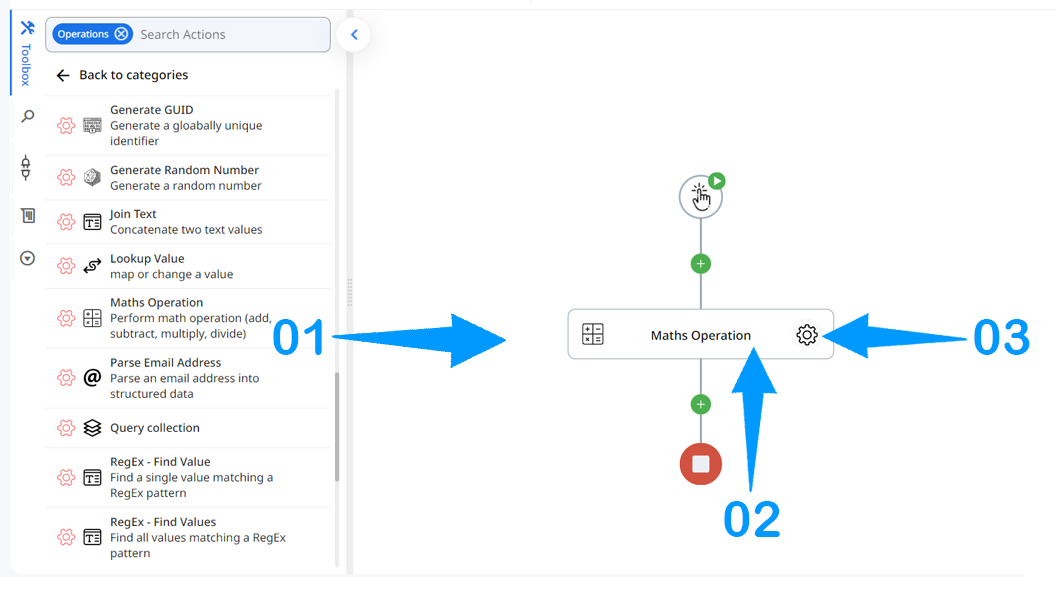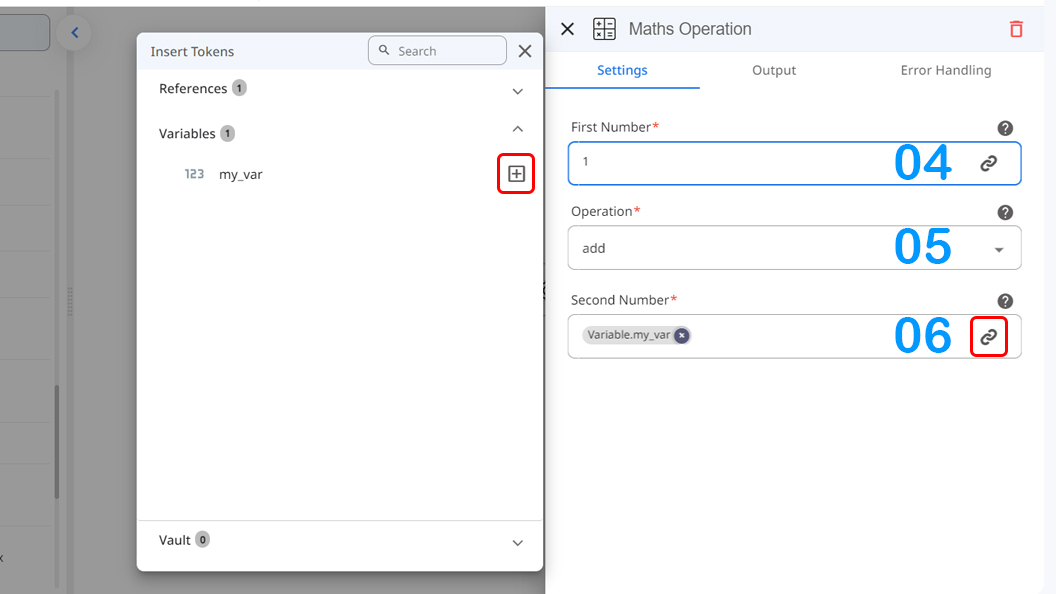# Usage

This action is for mathematical operations that utilized to perform Four main calculations on given numbers or variables.

# Fields

1.First Number - The first number for the mathematical operation.
2.Operation - Four main math operations.
3.Second Number - The second number for the mathematical operation.

# Demonstration on how to use it in a flow

1.Drag and drop the Maths Operations action into the flow.

2.Set the name of your choice or let it be as default

3.Click the gear icon to open its settings.4.Enter the first number of operation or use token picker to choose from other actions output or a numeric variable.
5.choose one of four main math operations such as add or subtract.
6.Enter the second number of operation or use token picker to choose from other actions output or a numeric variable.🚧

Caution:

If you use a variable as one or both of the operation numbers, remember that the Maths Operation does not change the value of variable(s) by default .
In order to change the value you have to use Set Variable action and set Maths Operation action's output as the new value.